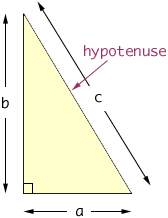Name: Phillipe Who is asking: Student Question: how do you find the hypotenuse of a right triangle? Hi Phillipe, One of the most famous theorems on mathematics is the Theorem of Pythagoras. This theorem tells you the relationship among the three sides of a right triangle. In a right triangle, the side opposite the right angle is called the hypotenuse.If the lengths of the sides of a right triangle are a, b and c where c is the length of the hypotenuse as in the diagram above, then the Theorem of Pythagoras says that a2 + b2 = c2 Thus, for example if a = 5 and b = 12 then c2 = 52 + 122 = 25 + 144 = 169 and hence c = 13. Cheers, Penny Go to Math Central